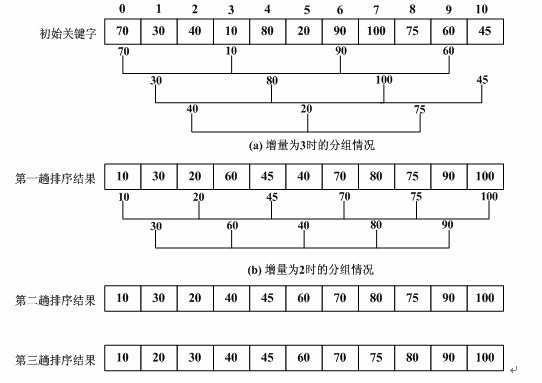# Javascript算法——希尔排序

footballboy

0收藏

1. 算法介绍

1.1 算法描述

• 插入排序在对几乎已经排好序的数据操作时，效率高，即可以达到线性排序的效率；
• 但插入排序一般来说是低效的，因为插入排序每次只能将数据移动一位；
希尔排序的基本思想是：先将整个待排序的记录序列分割成为若干子序列分别进行直接插入排序，待整个序列中的记录基本有序时，再对全体记录进行依次直接插入排序。1.2 算法步骤

1.3 算法实现

``````function shellSort(arr) {
var len = arr.length,
temp,
gap = 1;
while(gap < len/3) {          //动态定义间隔序列
gap = gap*3+1;
}
for (gap; gap > 0; gap = Math.floor(gap/3)) {
for (var i = gap; i < len; i++) {
temp = arr[i];
for (var j = i-gap; j >= 0 && arr[j] > temp; j -= gap) {
arr[j+gap] = arr[j];
}
arr[j+gap] = temp;
}
}
return arr;
}
``````帖子
视频
声望
粉丝
最近发布
热门推荐
社区精华内容